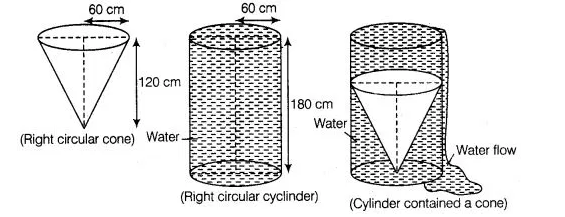# A solid right circular cone of height 120 cm

Question:

A solid right circular cone of height 120 cm and radius 60 cm is placed in a right circular cylinder full of water of height 180 cm. Such that it touches the

bottom. Find the volume of water left in the cylinder, if the radius of the cylinder is equal to the radius to the cone.Solution:

(i)   Whenever we placed a solid right circular cone in a right circular cylinder with full of water, then volume of a solid right circular cone is equal to

the volume of water failed from the cylinder.

(ii)  Total volume of water in a cylinder is equal to the volume of the cylinder.

(iii) Volume of water left in the cylinder = Volume of the right circular cylinder – volume of a

right circular cone.

Now, given that

Height of a right circular cone = 120 cm

Radius of a right circular cone = 60 cm

$\therefore$ Volume of a right circular cone $=\frac{1}{3} \pi r^{2} \times h$

$=\frac{1}{3} \times \frac{22}{7} \times 60 \times 60 \times 120$

$=\frac{22}{7} \times 20 \times 60 \times 120$

$=144000 \pi \mathrm{cm}^{3}$

$\therefore$ Volume of a right circular cone $=$ Volume of water falled from the cylinder

$=144000 \pi \mathrm{cm}^{3}$ [from point (i)]

Given that, height of a right circular cylinder $=180 \mathrm{~cm}$

and radius of a right circular cylinder $=$ Radius of a right circular cone

$=60 \mathrm{~cm}$

$\therefore$ Volume of a right circular cylinder $=\pi r^{2} \times h$

$=\pi \times 60 \times 60 \times 180$

$=648000 \pi \mathrm{cm}^{3}$

So, volume of a right circular cylinder $=$ Total volume of water in a cylinder

$=648000 \pi \mathrm{cm}^{3}$ [from point (ii)]

From point (iii),

Volume of water left in the cylinder

$=$ Total volume of water in a cylinder - Volume of water falled from

the cylinder when solid cone is placed in it

$=648000 \pi-144000 \pi$

$=504000 \pi=504000 \times \frac{22}{7}=1584000 \mathrm{~cm}^{3}$

$=\frac{1584000}{(10)^{6}} m^{3}=1.584 m^{3}$

Hence, the required volume of water left in the cylinder is $1.584 \mathrm{~m}^{3}$.